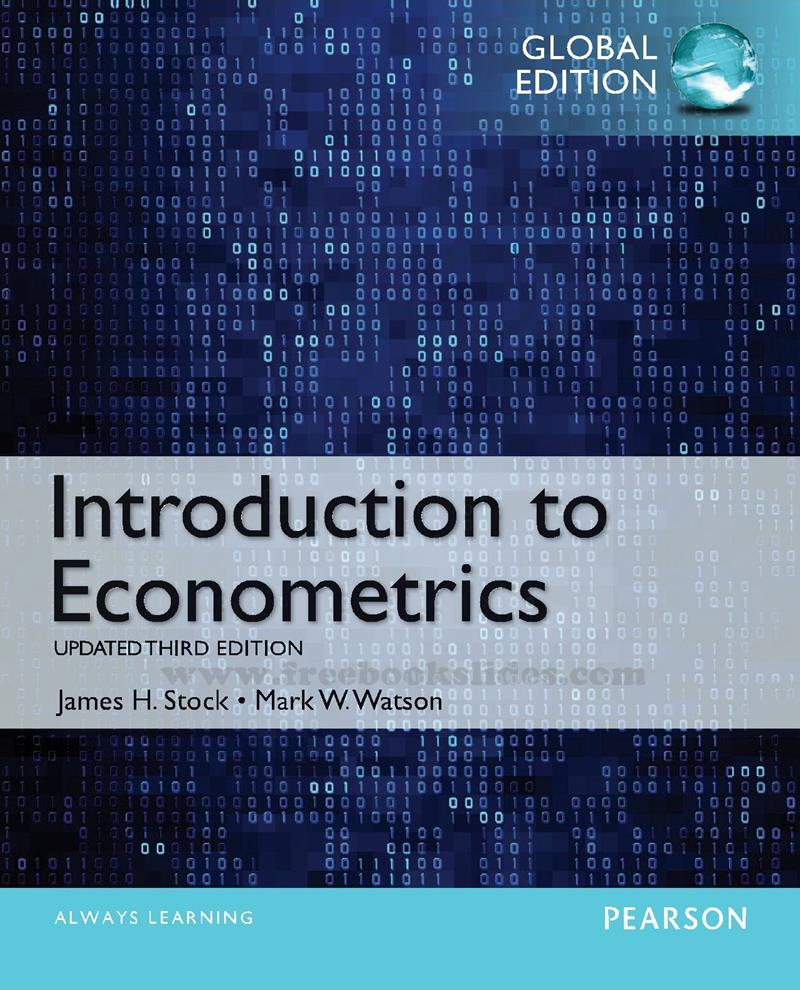### Ebook Introduction to Econometrics 3e by Stock and Watson (Global Edition)Introduction to Econometrics
3rd Edition (Global Edition)
by James H. Stock • Mark W. Watson
Pe*rson Educ*tion / Pr*ntice Hall
PDF 14.0 MB

PART ONE Introduction and Review
Chapter 1 Economic Questions and Data 47
Chapter 2 Review of Probability 60
Chapter 3 Review of Statistics 111
Part Two Fundamentals of Regression Analysis
Chapter 4 Linear Regression with One Regressor 155
Chapter 5 Regression with a Single Regressor: Hypothesis Tests and Confidence
Intervals 192
Chapter 6 Linear Regression with Multiple Regressors 228
Chapter 7 Hypothesis Tests and Confidence Intervals in Multiple Regression 263
Chapter 8 Nonlinear Regression Functions 302
Chapter 9 Assessing Studies Based on Multiple Regression 361
Part Three Further Topics in Regression Analysis
Chapter 10 Regression with Panel Data 396
Chapter 11 Regression with a Binary Dependent Variable 431
Chapter 12 Instrumental Variables Regression 470
Chapter 13 Experiments and Quasi-Experiments 521
Part Four Regression Analysis of Economic Time Series Data
Chapter 14 Introduction to Time Series Regression and Forecasting 568
Chapter 15 Estimation of Dynamic Causal Effects 635
Chapter 16 Additional Topics in Time Series Regression 684
Part Five The Econometric Theory of Regression Analysis
Chapter 17 The Theory of Linear Regression with One Regressor 722
Chapter 18 The Theory of Multiple Regression 751